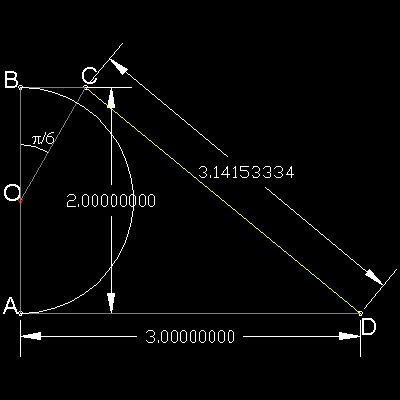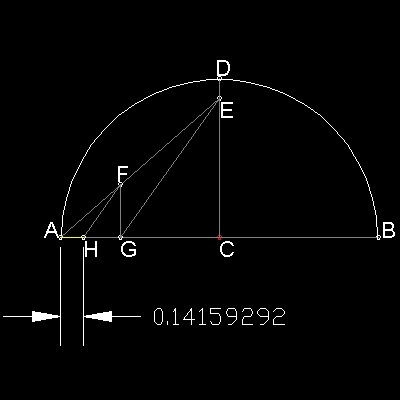Approximation
Go to Fun_Math Content Table   Three Famous Problems   Greek Circle Squarer   Later Squarer

## Approximation

### 1. Kochansky

Kochansky published this result in 1685.#### Construction steps

OA = OB = r, and AD = 3r

BC is paralllel to AD, and angle BOC = 30 degrees.

Then CD = 3.141533..
*************** Kochansky.dwg ***************

To create this drawing :
Then from command line, type Kochansky

### 2. Gelder

Jacob de Gelder (1765- 1848) published this result in 1849.
This is based on the approximation 355/113 = 3 + 42/(72 + 82).
This decimal portion is to be constructed.#### Construction steps

CD = 1, CE = 7/8, AF = 1/2

FG is parallel to CD, and HF is parallel to GE.

Then AH = 42/(72 + 82).

**************** Gelder.dwg *****************

To create this drawing :
Then from command line, type Gelder

### 3. Ernest William Hobson (1856 - 1933)

Hobson published this result in 1913. He constructed the approximate value of square root of pi,which is the true "circle squaring". p1/2 = 1.77245... His constructed length is 1.77246...,which is very,very close!!#### Construction steps

OA = 1.0, OD = 3/5, OF = 3/2, and OE = 1/2

Draw the semicircles DGE , AHF with DE and AF as diameters.

The perpendicular to AB through O intersects These semicircles at G & H.

Then GH = 1.77246...

**************** Hobson.dwg *****************

To create this drawing :
Then from command line, type Hobson